# 2-diode equation¶

This is the simplest method for simulating the behaviour of a solar cell, using electrical components to model the different transport and recombination mechanisms of the device. The 2D model is widely applied when modelling solar cells at the most engineering end of the topic, when a detailed knowledge of the solar cell structure (layers, absorption coefficient, etc.) are not known or sought. It is often used to fit experimental IV curves and find approximate, general information on the solar cell quality without entering on the fundamental processes. It can provide valuable information to engineers, when designing solar modules for example, or for diagnostic purposes The complete form of the equation is: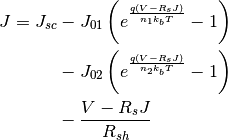Generally, the photocurrent is modelled as a current source (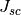), with radiative and non-radiative recombination modelled as two diodes with reverse saturation currents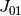and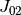, and ideality factors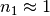and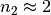, respectively. The shunt resistance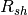accounts for alternative current paths between the contacts of the solar cell, being infinite in the ideal case, and the series resistance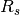accounts for the other transport losses. The values of the saturation currents and ideality factors can, ultimately, be calculated from the material properties and device structure, as is done in the depletion approximation model, but the 2D model allows them to be provided directly as input, obtained from a fit to experimental data, for example. They can also be calculated internally, using the DB solver to obtainand, and then using a radiative efficiency coefficient to obtain. The radiative efficiency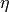is defined as the fraction of radiative current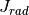at a given reference total current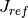: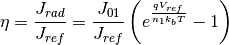The reference voltage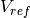can be written as a function ofandas: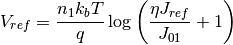On the other hand, the radiative coefficient can also be written as: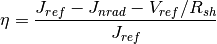Combining the last two equations and using the expression for the diode with ideality factor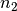,can be written as: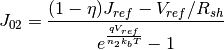In the common situation of very large shunt resistance and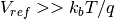, this equation further simplifies to: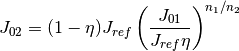This process can, of course, be reversed to use knowledge ofandat a given reference current to calculate the radiative efficiency of a solar cell, which is useful to compare different materials, technologies or processing methods. This was done by Chan et al. using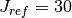mA/cm, obtainingvalues of 20% for InGaP, 22% for GaAs, and 27% for InGaAs devices (). It should be pointed out that this method is only valid under the assumption thatcorresponds only to radiative recombination andonly to non-radiative recombination, which is generally true for QW solar cells and some III-V solar cells, like those made of GaAs or InGaP, but not for Si or Ge, for example. Other definitions of the radiative efficiency are based on the external quantum efficiency, the Iand Vof the cell, as described by Green (2011) ().

Despite the simplicity of the 2-diode model, it is very useful to guide the design of new solar cells and explore the performance of new materials, such as dilute bismuth alloys (), or to asses the performance of large arrays of solar cells ().

## 2-diode equation functions¶

solcore.analytic_solar_cells.diode_equation.iv_2diode(junction, options)[source]

Calculates the IV curve of a junction object using the 2-diode equation. All parameters needed for the calculation need to be included in the junction object. Series resistance is included at solar cell level, not at junction level. The junction is then updated with an “iv” function that calculates the IV curve at any voltage.

Parameters: junction – A junction object. options – Solver options. None.
solcore.analytic_solar_cells.diode_equation.calculate_J01(Eg_in_eV, T, n)[source]

Calculate the reverse saturation current J01, assumed radiative, considering an absorption equal to 1 above the bandgap. Light trapping is included by considering the refractive index of the material: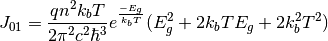Parameters: Eg_in_eV – Bandgap in eV T – Cell temperature n – Refractive index of the material The reverse saturation current J01
solcore.analytic_solar_cells.diode_equation.calculate_J02_from_Voc(J01, Jsc, Voc, T, R_shunt=1000000000000000.0)[source]

Calculates J02 based on the J01, Jsc and the Voc. It is just the result of solving the 2-diode equation for J02. Ideality factors n1 and n2 are assumed to be equal to 1 and 2, respectively.

Parameters: J01 – Reverse saturation current J01, typically the radiative component Jsc – Short circuit current (=photocurrent) Voc – Open circuit voltage T – Temperature R_shunt – Shunt resistance (default = 1e15) The reverse saturation current J02
solcore.analytic_solar_cells.diode_equation.calculate_J02_from_rad_eff(J01, radiative_efficiency, V, T, R_shunt=1000000000000000.0)[source]

Calculates J02 based on J01 and a radiative efficiency at a given voltage and temperature. Ideality factors n1 and n2 are assumed to be equal to 1 and 2, respectively.

Parameters: J01 – Reverse saturation current J01, typically the radiative component radiative_efficiency – Fraction of the dark current that is radiative V – Operating voltage T – Temperature R_shunt – Shunt resistance (default = 1e15)
solcore.analytic_solar_cells.diode_equation.calculate_j02_from_J01_Jsc_reference_radiative_efficiency(J01, Jsc_ref, radiative_efficiency, T)[source]
solcore.analytic_solar_cells.diode_equation.update_j0(junctions, T, Tref)[source]

Updates the reverse saturation currents for the target temperature knowing their values at a reference temperature.

Parameters: junctions – List of junctions T – Target temperature Tref – Working temperature List of junctions with their saturation currents updated for the new temperatures